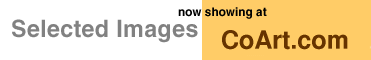[ Graphics Archive | Up | Comments ]

### Special Topics:Computational Geometry

Halfspace intersection with Qhull by Brad BarberA halfspace is all points to one side of a plane. The intersection of a set of halfspaces is a convex set. A related area is linear programming. The output of a linear program is a vertex of a halfspace intersection.

This Geomview picture from Qhull illustrates how to compute a halfspace intersection with the convex hull. The cone with the small square facets is the convex hull of two cospherical polygons and an apex. The other cone is the dual of the first. Each vertex of the second cone corresponds to a facet or halfspace of the first. Each facet of the second cone corresponds to a vertex or halfspace intersection of the first. For example, a vertex with four edges corresponds to a facet with four neighbors.

Assume the origin is inside the cone and let the first cone's facets define a set of halfspaces. To compute a dual point divide a facet's coefficients by its offset. The second cone is the convex hull of the dual points. The dual points for the second cone's facets are the vertices of the first cone. The vertices are the intersection of the first cone's facets.

Qhull uses floating point arithmetic. The non-triangular facets were merged by Qhull. For halfspace intersection, this is needed when more than three planes meet at the same point.

How to make it: `rbox 10 r s Z1 G0.3 | qhull Qx FV n | qhull H Qx G >a`

Image created: April 1995The Geometry Center Home Page